In: Physics

# The intensity of an earthquake wave passing through the Earth is measured to be 3.0 X...

The intensity of an earthquake wave passing through the Earth is measured to be 3.0 X 106 J/m2 s at a distance of 54 km from the source.

(a) What was its intensity when it passed a point only 1.0 km from the source?

(b) At what rate did energy pass through an area of 2.0m2 at 1.0 km?

## Solutions

##### Expert Solution

Concepts and reason

The concepts used to solve this problem are the relation between the distance and the intensity of earth quake wave, and the relation between rate of energy that passes and the intensity of the earth quake wave.

First, use the relation between the distance and the intensity of the earth quake wave to find the intensity of the earth quake wave at a distance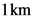from the source point.

Then, use the relation between rate of energy that passes and the intensity of the earth quake wave to find the rate of energy that passes through an area of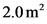at a distance offrom the source position.

Fundamentals

The expression that relates the distance and intensity of the earth quake wave is as follows: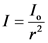Here, the intensity at a distance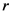from the source is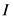and the intensity of the source is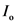.

The expression that relates the rate of energy that passes and intensity of the earth quake wave is given by the relation as follows: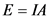Here, the energy is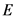and the area through which the energy passes is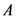.

(a)

The expression that relates the distance and intensity of the earth quake wave is as follows:Let the intensity at a distance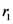be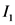and the intensity at a distancebe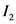.

Therefore, the expression of intensitiesandare as follows: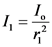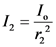Now, take the ratio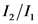.Rearrange to get.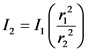Substitute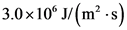for,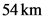for, andforto find the intensity at a distancefrom the source.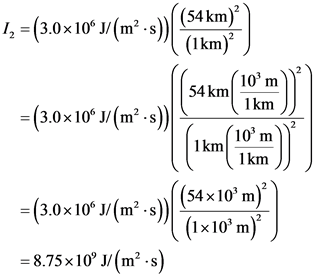(b)

The expression that relates the rate of energy that passes and the intensity of the earth quake wave is given by the relation as follows:Substitute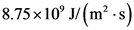forandforin the above expression to find the rate of energy that passes through the area ofat a distance of.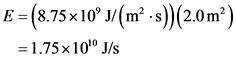Ans: Part a

The intensity at a distance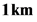from the source is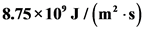.

Part b

The rate of energy that passes through the area of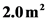atdistance is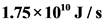.

## Related Solutions

##### The intensity of an earthquake wave passing through the Earth is measured to be 3.0 X...
The intensity of an earthquake wave passing through the Earth is measured to be 3.0 X 106 J/m2 s at a distance of 54 km from the source. (a) What was its intensity when it passed a point only 1.0 km from the source? (b) At what rate did energy pass through an area of 2.0m2 at 1.0 km?
##### The intensity of an earthquake wave passing through the Earth is measured to be 2.5×106 J/(m2⋅s)...
The intensity of an earthquake wave passing through the Earth is measured to be 2.5×106 J/(m2⋅s) at a distance of 47 km from the source. What was its intensity when it passed a point only 1.0 kmkm from the source? Express your answer to two significant figures and include the appropriate units. At what rate did energy pass through an area of 4.0 m2m2 at 1.0 kmkm ? Express your answer to two significant figures and include the appropriate units.
##### An earthquake P wave traveling at 7.7km/s strikes a boundary within the Earth between two kinds...
An earthquake P wave traveling at 7.7km/s strikes a boundary within the Earth between two kinds of material. If it approaches the boundary at an incident angle of 44? and the angle of refraction is 33?, what is the speed in the second medium? Express your answer to two significant figures and include the appropriate units.
##### A polarized light wave passes through a polarizing filter which reduced the intensity of the light...
A polarized light wave passes through a polarizing filter which reduced the intensity of the light to 25% of the incident intensity. What would the intensity become if the incident light is unpolarized? PLEASE EXPLAIN :))
##### The Richter scale is a measure of the intensity of an earthquake. The energy E (in joules) released by the quake
The Richter scale is a measure of the intensity of an earthquake. The energy E (in joules) released by the quake is related to the magnitude M on the Richter scale as follows. E = 104.4101.5M How much more energy is released by a magnitude 7.6 quake than a 5.6 quake?
##### The Current method for 100% detection of contraband passing through X-Ray screeners by TSA agents in...
The Current method for 100% detection of contraband passing through X-Ray screeners by TSA agents in the United is 14%. We develop a new way that teaches screeners to use different color schemes to illuminate items such that a person can see the items in the color scheme that makes sense to them. Out of the 24 TSA agents that volunteered to participate in a program, 8 were now capable of 100% detection. Perform an appropriate hypothesis test to determine...
##### Sound is passing perpendicularly through an open window whose dimensions are 1.1 m x 0.75 m....
Sound is passing perpendicularly through an open window whose dimensions are 1.1 m x 0.75 m. The sound intensity level is 83 dB above the threshold of human hearing. How much sound energy comes through the window in one hour?
##### 1. a) You have a maximum magnetic field of 1.45 x 10^(-5) Tesla when measured 3.0...
1. a) You have a maximum magnetic field of 1.45 x 10^(-5) Tesla when measured 3.0 cm away from the long section of wire. What is the maximum current that must flow through the wire to create this B-field? b) You want to make sure you clearly see the time dependence on your phone’s app, decide to design the RC portion of your circuit so that it takes 1.0 seconds for the current to decrease to 10.% of its maximum...
##### Two particles A and B are identical except that in passing through matter A interacts with...
Two particles A and B are identical except that in passing through matter A interacts with a force that is stronger than the one with which B interacts. Which of the following is true when comparing what happens when these particles pass through some material? A. On average, A will penetrate through more material before interacting than B. B. On average, A will penetrate through less material before interacting than B. C. On average, A and B will penetrate through...
##### Molten ZnBr2 is electrolyzed by passing a current of 15.0 A through an electrolysis cell for...
Molten ZnBr2 is electrolyzed by passing a current of 15.0 A through an electrolysis cell for a certain length of time. In this process, 45.75g Br2 are deposited_________( anode or cathode). How long does this process require? What is the chemical reaction taking place at the other electrode? What mass of the second product is released at the other electrode?